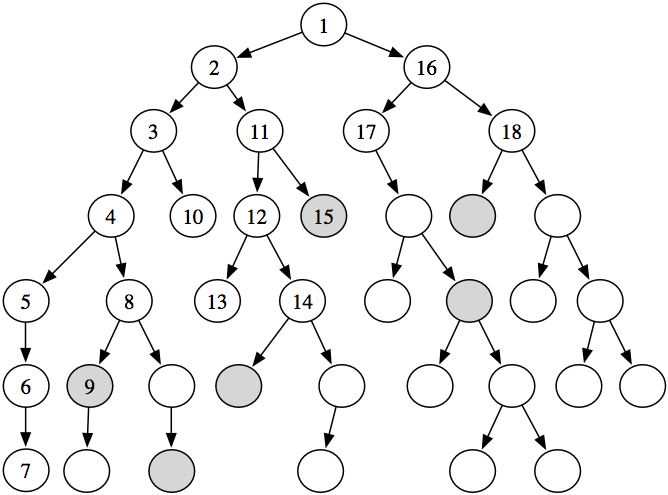### 3.7.4 Branch and Bound

Depth-first branch-and-bound search is a way to combine the space saving of depth-first search with heuristic information. It is particularly applicable when many paths to a goal exist and we want an optimal path. As in A* search, we assume that h(n) is less than or equal to the cost of a lowest-cost path from n to a goal node.

The idea of a branch-and-bound search is to maintain the lowest-cost path to a goal found so far, and its cost. Suppose this cost is bound. If the search encounters a path p such that cost(p)+h(p) ≥ bound, path p can be pruned. If a non-pruned path to a goal is found, it must be better than the previous best path. This new solution is remembered and bound is set to the cost of this new solution. It then keeps searching for a better solution.

Branch-and-bound search generates a sequence of ever-improving solutions. Once it has found a solution, it can keep improving it. Branch-and-bound search is typically used with depth-first search, where the space saving of the depth-first search can be achieved. It can be implemented similarly to depth-bounded search, but where the bound is in terms of path cost and reduces as shorter paths are found. The algorithm remembers the lowest-cost path found and returns this path when the search finishes.

1: Procedure DFBranchAndBound(G,s,goal,h,bound0)
2:           Inputs
3:                     G: graph with nodes N and arcs A
4:                     s: start node
5:                     goal: Boolean function on nodes
6:                     h: heuristic function on nodes
7:                     bound0: initial depth bound (can be if not specified)
8:           Output
9:                     a least-cost path from s to a goal node if there is a solution with cost less than bound0
10:                     or if there is no solution with cost less than bound0
11:           Local
12:                     best_path: path or
13:                     bound: non-negative real
14:                     Procedure cbsearch(⟨n0,...,nk)
15:                               if (cost(⟨n0,...,nk⟩)+h(nk) < bound) then
16:                               if (goal(nk)) then
17:                                         best_path ←⟨n0,...,nk
18:                                         bound ←cost(⟨n0,...,nk⟩)
19:                               else
20:                                         for each arc ⟨nk,n⟩∈A do
21:                                                   cbsearch(⟨n0,...,nk,n⟩)
22:           best_path ←⊥
23:           bound ←bound0
24:           cbsearch(⟨s⟩)
25:           return best_path
Figure 3.11: Depth-first branch-and-bound search

The algorithm is shown in Figure 3.11. The internal procedure cbsearch, for cost-bounded search, uses the global variables to provide information to the main procedure.

Initially, bound can be set to infinity, but it is often useful to set it to an overestimate, bound0, of the path cost of an optimal solution. This algorithm will return an optimal solution - a least-cost path from the start node to a goal node - if there is a solution with cost less than the initial bound bound0.

If the initial bound is slightly above the cost of a lowest-cost path, this algorithm can find an optimal path expanding no more arcs than A* search. This happens when the initial bound is such that the algorithm prunes any path that has a higher cost than a lowest-cost path; once it has found a path to the goal, it only explores paths whose the f-value is lower than the path found. These are exactly the paths that A* explores when it finds one solution.

If it returns when bound0=∞, there are no solutions. If it returns when bound0 is some finite value, it means no solution exists with cost less than bound0. This algorithm can be combined with iterative deepening to increase the bound until either a solution is found or it can be shown there is no solution. See Exercise 3.13.Figure 3.12: The nodes expanded in depth-first branch-and-bound search

Example 3.17: Consider the tree-shaped graph in Figure 3.12. The goal nodes are shaded. Suppose that each arc has length 1, and there is no heuristic information (i.e., h(n)=0 for each node n). In the algorithm, suppose depth0=∞ and the depth-first search always chooses the leftmost child first. This figure shows the order in which the nodes are checked to determine if they are a goal node. The nodes that are not numbered are not checked for being a goal node.

The subtree under the node numbered "5" does not have a goal and is explored fully (or up to depth depth0 if it had a finite value). The ninth node checked is a goal node. It has a path cost of 5, and so the bound is set to 5. From then on, only paths with a length of less than 5 are checked for being a solution. The fifteenth node checked is also a goal. It has a path cost of 3, and so the bound is reduced to 3. There are no other goal nodes found, and so the path to the node labeled 15 is returned. It is an optimal path. There is another optimal path that is pruned; the algorithm never checks the children of the node labeled with 18.

If there was heuristic information, it could be used to prune parts of the search space, as in A* search.